Search Results
• #### find Consider the Transfer Function Shown Below: G(S) = (s +2) s(s + 3)(s + 5)2...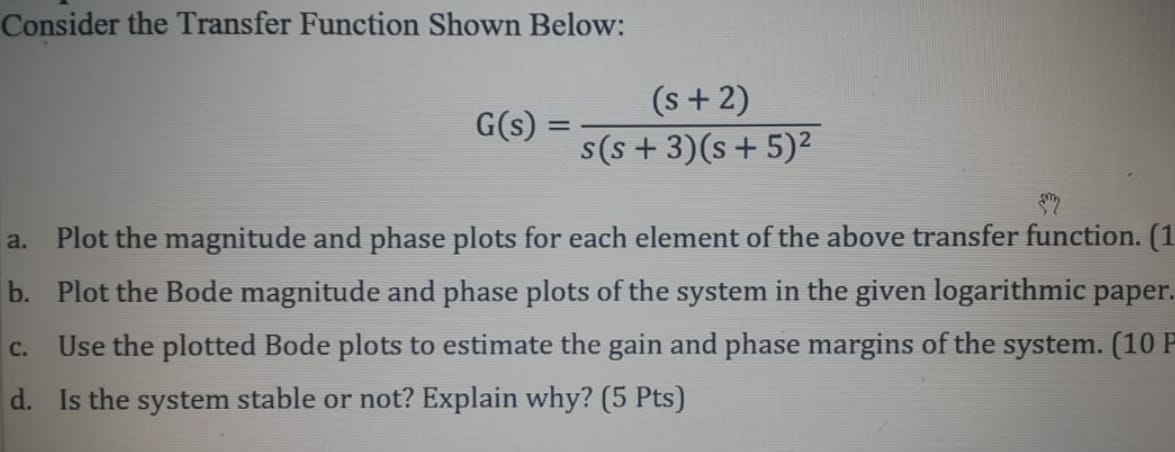find Consider the Transfer Function Shown Below: G(S) = (s +2) s(s + 3)(s + 5)2 a. Plot the magnitude and phase plots for each element of the above transfer function. (1 b. Plot the Bode magnitude and phase plots of the system in the given logarithmic paper. Use the plotted Bode plots to estimate the gain and phase margins...

• #### (IV) Consider the following block diagram W (s) Y (s) E(s) C1 (s) R (s) P...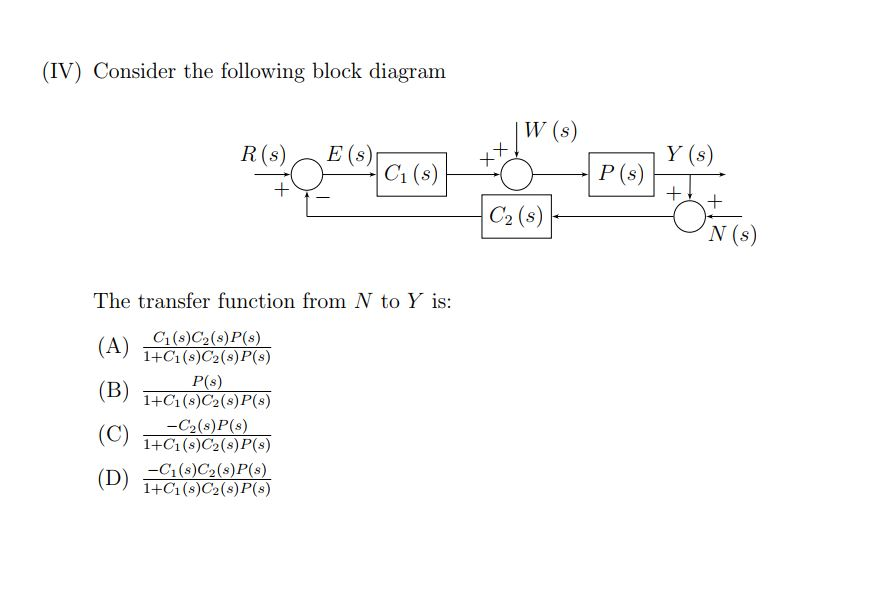(IV) Consider the following block diagram W (s) Y (s) E(s) C1 (s) R (s) P (s) + C2 (s) N (s) The transfer function from N to Y is: C1(s)C2(s)P(s) 1+C1(s)C2(s)P(s) (A) P(s) 1+C1 (s)C2(s)P(s) -C2(s) P(s) (C) 1+C1(s)C2(s)P(s) (B) -C1(s)C2(s)P(s) 1+C1(s)C2(s)P(s) (D)

• #### Given the systems below, sketch the root locus: R(S) K C(s) s(s+2)(s+5)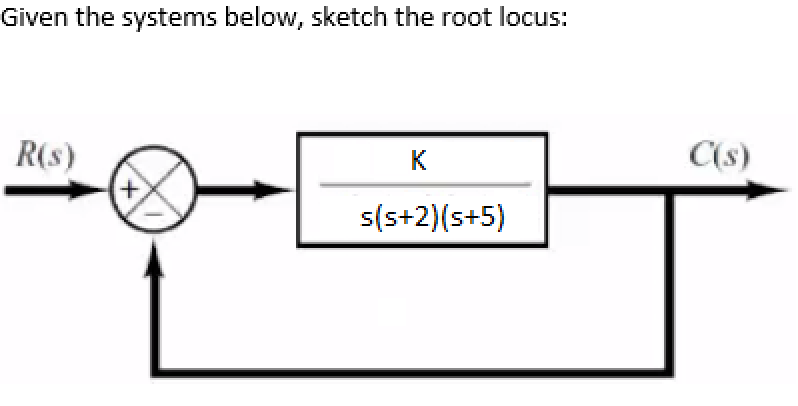Given the systems below, sketch the root locus: R(S) K C(s) s(s+2)(s+5)

• #### A transfer function is given by H(s) 2(s +1) (s+2)(s + 10) where s= jw. References...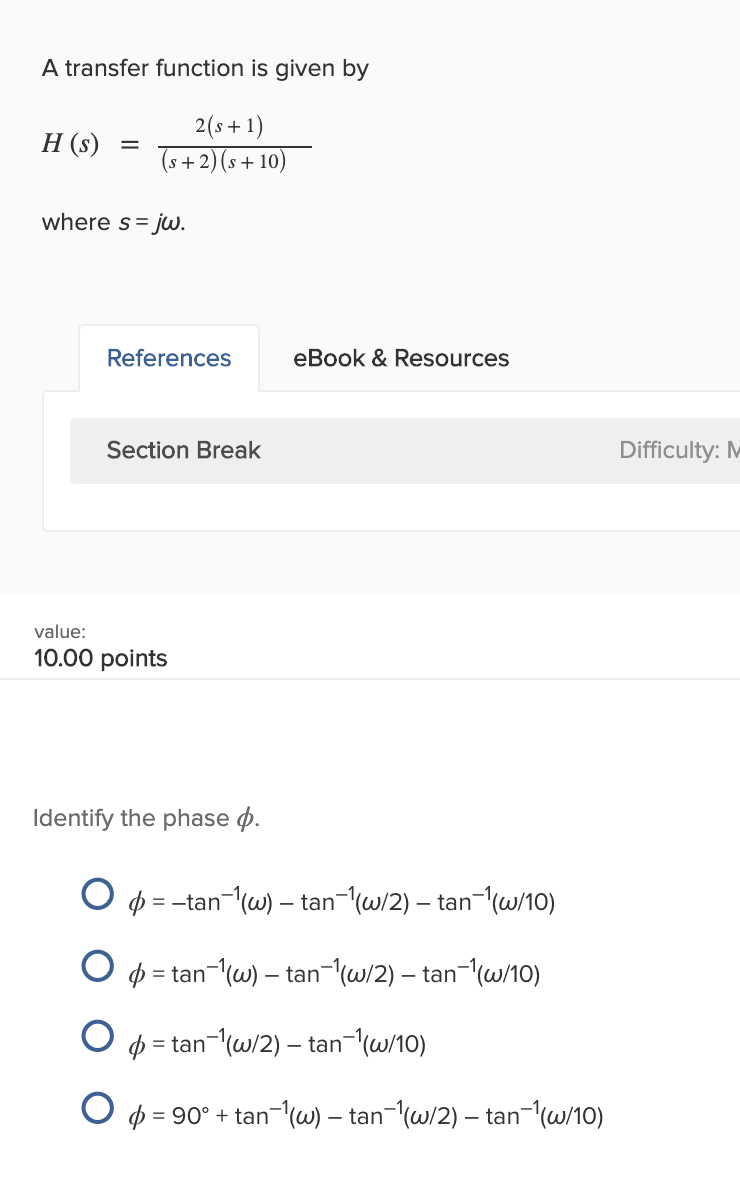A transfer function is given by H(s) 2(s +1) (s+2)(s + 10) where s= jw. References eBook & Resources Section Break Difficulty: M value: 10.00 points Identify the phase o. p = -tan-'(W) – tan-|(w/2) – tan-|(w/10) O p = tan-1W) – tan-1(w/2) – tan-1(w/10) O p = tan-'w/2) – tan-'(w/10) O 0 = = 90° + tan-'(W) – tan-'w/2)...

• #### balance this equ Mg(s) + N2(g) + O2(g) → MgO(s) + Mg3N2(s) MgO(s) + Mg3N2(s) +...

balance this equ Mg(s) + N2(g) + O2(g) → MgO(s) + Mg3N2(s) MgO(s) + Mg3N2(s) + H2O(l) → MgO(s) + Mg(OH)2 (s) + NH3(g) MgO(s) + Mg(OH)2 (s) → MgxOy(s) + H2O(g)

• #### Fe2O3(s) + 2Al(s)Al2O3(s) + 2Fe(s) for which H° = -851.5 kJ and S° = -37.00 J/K...

Fe2O3(s) + 2Al(s)Al2O3(s) + 2Fe(s) for which H° = -851.5 kJ and S° = -37.00 J/K at 298.15 K. (1) Calculate the entropy change of the UNIVERSE when 2.413 moles of Fe2O3(s) react under standard conditions at 298.15 K. S universe =    J/K (2) Is this reaction reactant or product favored under standard conditions? ( 3) If the reaction...

• #### a) choices are 3 m/s/s, 1/3 m/s/s, 3 m/s, and -3 m/s. b) choices are 3/4...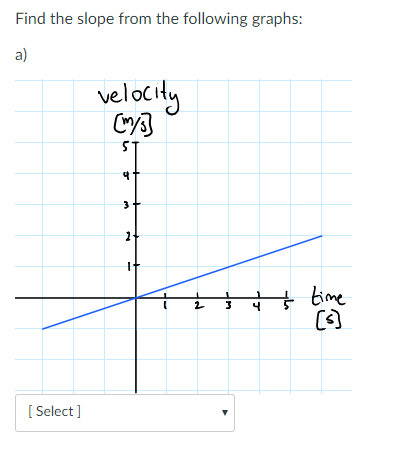a) choices are 3 m/s/s, 1/3 m/s/s, 3 m/s, and -3 m/s. b) choices are 3/4 m/s, -4/3 m/s/s, -3/4 m/s, 2 m/s. c) choices are 1 m/s, 5 m/s, -5 m/s, -1 m/s Find the slope from the following graphs: a) velocit Cy พา time [ Select ] b) [Select ] c) find the instantaneous slope at time t-2s...

• #### For the given system (s+25) P(s) s(s+1)(s+3000) 1. Sketch, by hand, the Bode asymptote plots for phase and magnitude. S...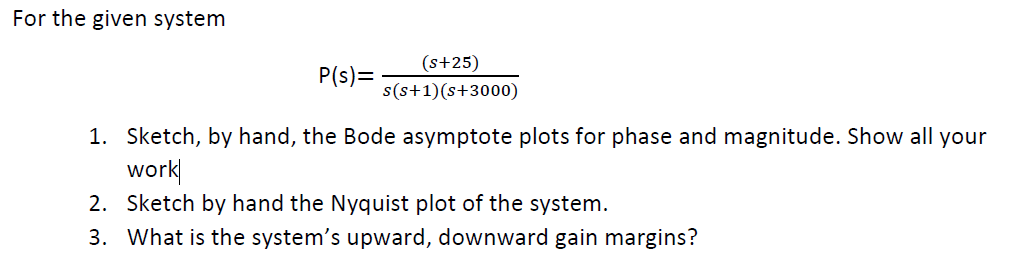For the given system (s+25) P(s) s(s+1)(s+3000) 1. Sketch, by hand, the Bode asymptote plots for phase and magnitude. Show all your work 2. Sketch by hand the Nyquist plot of the system 3. What is the system's upward, downward gain margins? For the given system (s+25) P(s) s(s+1)(s+3000) 1. Sketch, by hand, the Bode asymptote plots for phase and...

• #### 2. Nise(9.6) For a unity feedback system KG(s) (s 6) G(s) T(s) (s 2)(s3)(s 5) 1...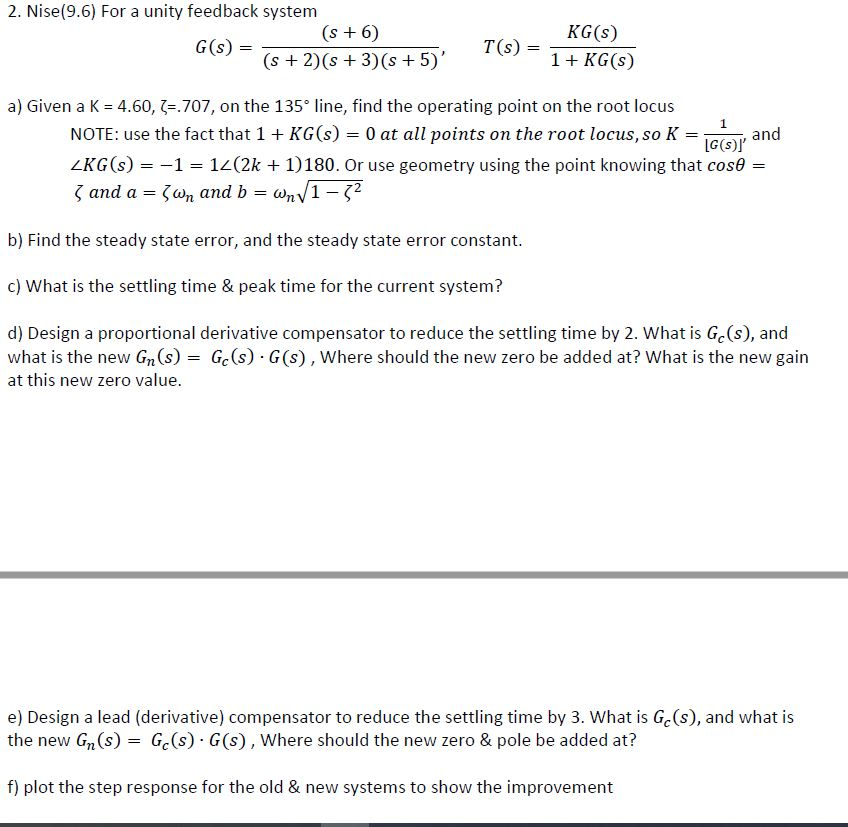2. Nise(9.6) For a unity feedback system KG(s) (s 6) G(s) T(s) (s 2)(s3)(s 5) 1 + KG(s) a) Given a K 4.60, .707, on the 135 line, find the operating point on the root locus NOTE: use the fact that 1 + KG(s) 0 at all points on the root locus, so K 1 and G(s)l 12(2k 1)180. Or...

• #### R(S) C(s) G(s) Figure P3 G(S) K(s2 – 2s + 2) s(s + 1)(8 +2) Problem...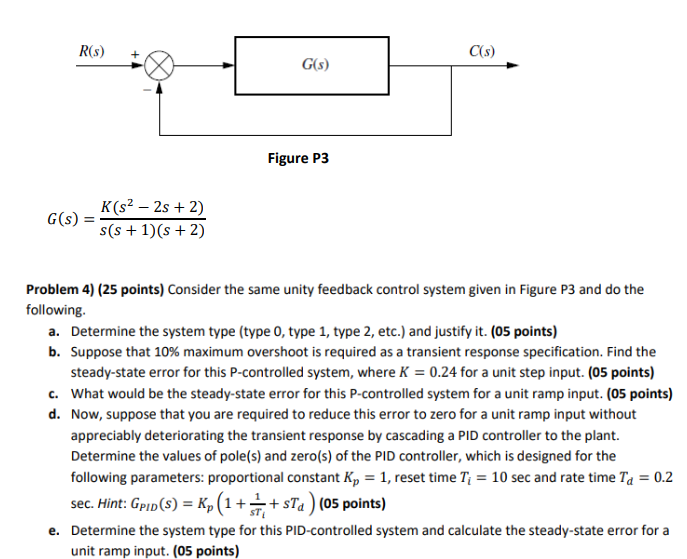R(S) C(s) G(s) Figure P3 G(S) K(s2 – 2s + 2) s(s + 1)(8 +2) Problem 4) (25 points) Consider the same unity feedback control system given in Figure P3 and do the following: a. Determine the system type (type 0, type 1, type 2, etc.) and justify it. (05 points) b. Suppose that 10% maximum overshoot is required as...